• er = rms_error(A1,A2) 这里A1，A2是原始数据和重构数据。 A1，A2的顺序无关紧要，互换它们也会得到相同的结果。matlab
• 矢量均方根误差 [Mse, Dse, stdL1, stdL2, alfa1]=RM_vec2rmse(uN, vN, uM, vM); ％ 输入% uN 是向量 N 的东向分量% vN 是向量 N 的北向分量％uM是向量M的向东分量% vM 是向量 M 的北向分量 ％ 输出% Mse 是系统...matlab
• matlab均方根误差

千次阅读 2019-01-08 15:02:00
Matlab均方根误差的计算 http://blog.sina.com.cn/s/blog_6210f654010308kv.html 转载于:https://www.cnblogs.com/moonlightml/p/10239041.html

Matlab均方根误差的计算

http://blog.sina.com.cn/s/blog_6210f654010308kv.html

转载于:https://www.cnblogs.com/moonlightml/p/10239041.html

展开全文• 本资源包含基于Matlab的rms方法计算矩阵、数组和向量元素的均方根值源码。 包含 实例1：创建一个向量（正弦波）并计算其均方根值 实例2：创建一个二维矩阵并计算其均方根值 实例3：计算二维矩阵沿指定维度的均方根值...
• RMSE评价算法，RMSE一般指均方根误差。均方根误差亦称标准误差。 RMSE（A，B）：A为原图，B为类比图
• 此函数参考原始信号计算信号的 RMSE（均方根误差）。 可以为 1-D/2-D/3-D 信号计算 RMSE。matlab
• 使用matlab分析一段声音信号的均方根

千次阅读 热门讨论 2020-02-22 07:12:13
均方根值（RMS）也称作为效值，它的计算方法是先平方、再平均、然后开方。本文给出了matlab实现它的代码，并不是直接调用函数，所以非常易读。

修改记录：2022年1月15日17:37:18。

感谢me141414，W.Scofieldm0_57478585等几位好友，帮我发现提供的源代码中会提示“未定义变量youxiao”的问题。经过作者测试，如果直接复制粘贴到matlab的m文件中，运行不起来。

初步猜测应该是matlab版本的问题， 作者2010版本上，这个youxiao仅仅是warning警告，不影响执行。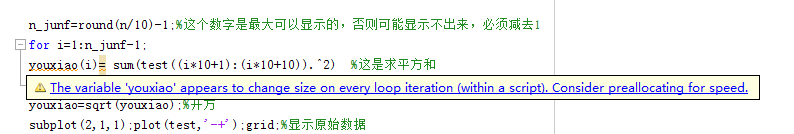解决的方法有两种：

第一种方法是将for语句后面的分号“;”删除，不过经过测试即使带着这个分号也是可以运行的，原因不详，大家可以讨论；

第二种方法是使用较低版本的matlab来执行。

第三种方法是向作者索取源代码和测试的txt数据，文末留有交流途径。

下面是正文

对一段声音信号（0.1秒采集一次）进行分析，用到了均方根。

先看结果，如下图所示，位于上方的是原始波形，一共1600多个点，位于下方的是均方根之后的波形，一共160多个点，每10个点取均方根。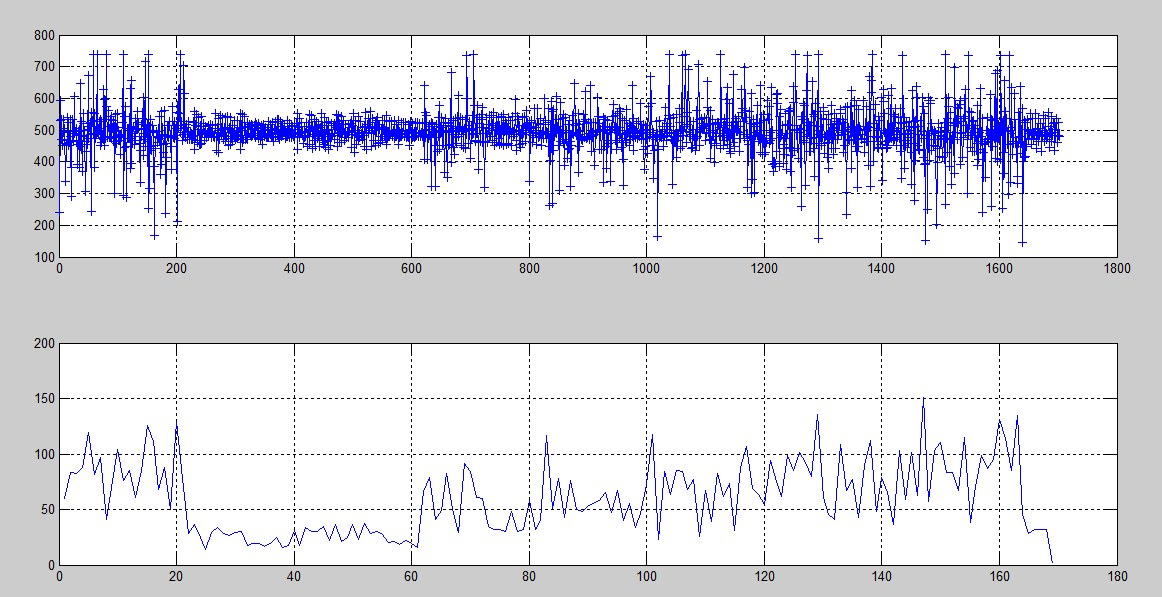什么是均方根，它的物理意义是什么？

均方根值（RMS）也称作为效值，它的计算方法是先平方、再平均、然后开方。如下图所示。它的物理意义是表达有效值比平均值更加合理，比如幅度为100V而占空比为0.5的方波信号，如果按平均值计算，它的电压只有50V，而按均方根值计算则有70.71V。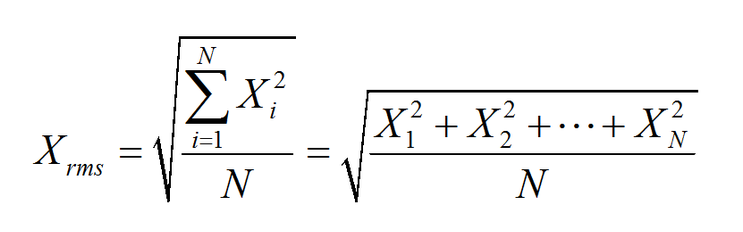算法分析：每隔固定的点数，将每个点求平方，然后都加起来，将累加之和除以点数，再开方。

下面使用matlab采用编程的方式实现这个过程

fid = fopen('e:/test/16.txt');%读取声音文件
format = '%d';%整数形式
y = fscanf(fid,format);%将文件内容放进数组
n=length(y);%获取数组长度
test= y-mean(y);%减去平均值

n_junf=round(n/10)-1;%这个数字是最大可以显示的，否则可能显示不出来，必须减去1
for i=1:n_junf-1;
youxiao(i)= sum(test((i*10+1):(i*10+10)).^2)  %这是求平方和
end;
youxiao=sqrt(youxiao);%开方
subplot(2,1,1);plot(test,'-+');grid;%显示原始数据

subplot(2,1,2);plot(youxiao);grid; %显示均方根数据

运行结果如下：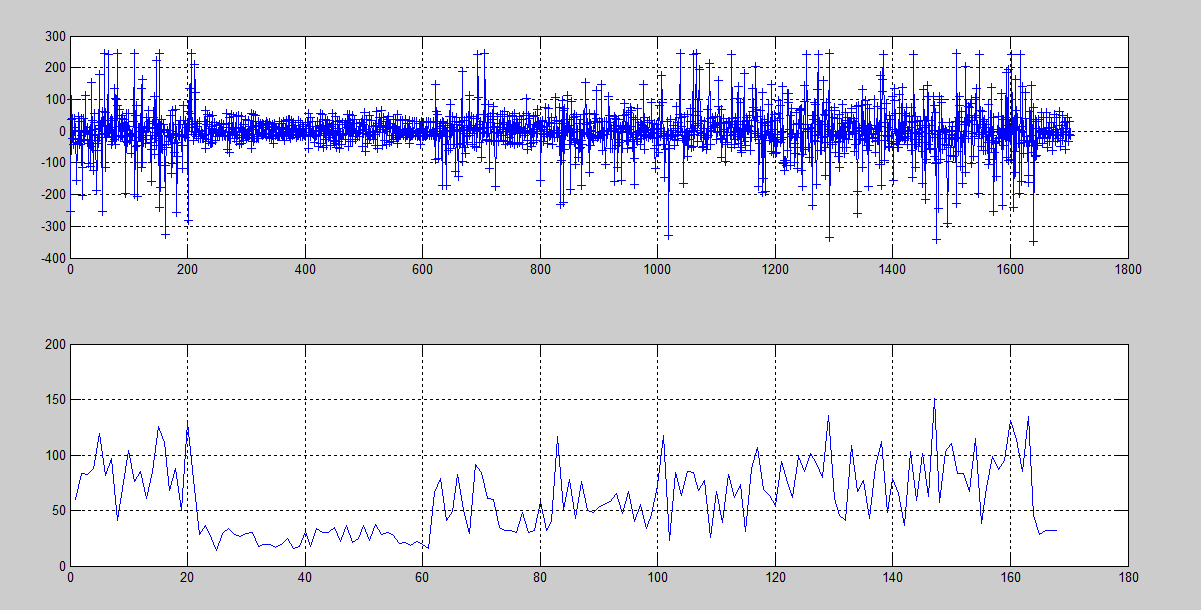进一步交流可通过以下方式展开全文matlab 均方根 RMS
• 1.matlab标准差STD的定义 S = std(A,w) 为上述任意语法指定...2.matlab均方根误差RMS的定义 S={[(x1-x)(x1-x) …(xn-x)(xn-x)]/N}^0.5（x为真值，N为样本个数）此公式中的X也就是所谓的真值，应改为x’1,x’2…（即真实

1.matlab标准差STD的定义
S = std(A,w) 为上述任意语法指定一个权重方案。当 w = 0 时（默认值），S 按 N-1 进行标准化。当 w = 1 时，S 按观测值数量 N 进行标准化。w 也可以是包含非负元素的权重矢量。在这种情况下，w 的长度必须等于 std 将作用于的维度的长度。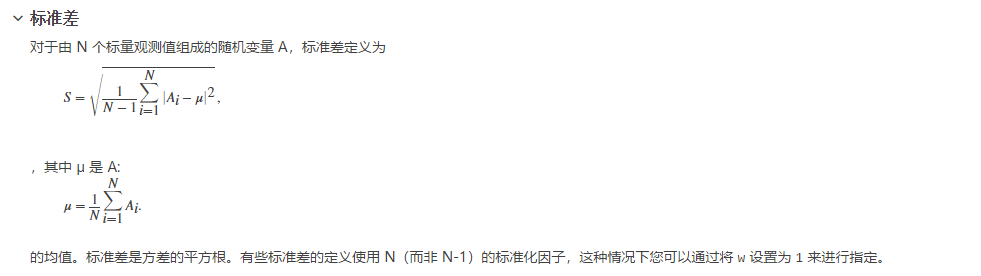2.matlab均方根误差RMS的定义
S={[(x1-x)(x1-x) …(xn-x)(xn-x)]/N}^0.5（x为真值，N为样本个数）此公式中的X也就是所谓的真值，应改为x’1,x’2…（即真实值）。均方根误差算的是观测值与其真值，或者观测值与其模拟值之间的偏差，而不是观测值与其平均值之间的偏差。
就是将所有输入的数据平方，然后除以数据总数，在进行开方
matlab中的rms这个函数和excel中的STDEVA函数一模一样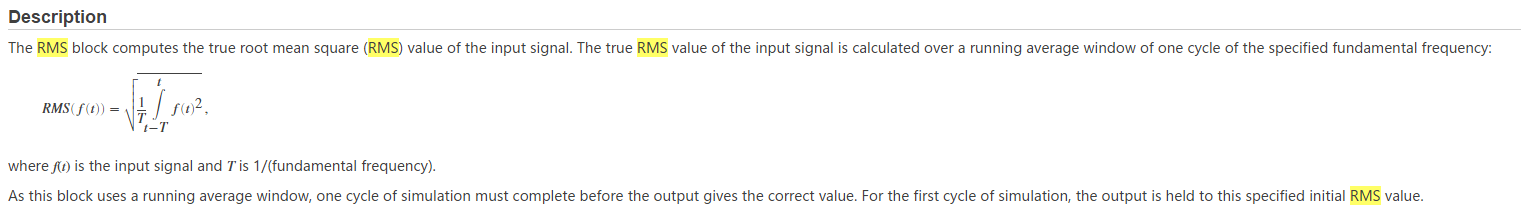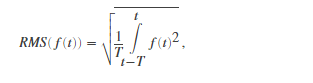3.两者区别：
1、均方差就是标准差（数学中的方差），标准差就是均方差（中误差）；
2、均方根误差不同于均方差；
3、均方根误差是各数据偏离真实值的距离平方和的平均数的开方。
均方差（标准差）是数据序列与均值的关系，而均方根误差是数据序列与真实值之间的关系。
因此，标准差是用来衡量一组数自身的离散程度，而均方根误差是用来衡量观测值同真值之间的偏差，它们的研究对象和研究目的不同，但是计算过程类似。

展开全文算法
• 小弟初学matlab今日师兄让偶识别一个信号的衰减。clearclc%%%%%%%%%%%%%%%%%%%%%%%%%%%%%% 一些参数 %%%%%%%%%%%%%%%%%%%%%%%%%%%%%%%%%%%%%%%%%%%%%%%%%%%%%%%%%%%%%%%%%%%%%%%%%%%%%%%%%%%%%%%%%%st=1024; ...

小弟初学matlab  今日师兄让偶识别一个信号的衰减。

clear

clc

%%%%%%%%%%%%%%%%%%%%%%%%%%%%%% 一些参数 %%%%%%%%%%%%%%%%%%%%%%%%%%%%%%%%%%%%%%%%%%%%%%%%%%%%%%%%%%%%%%%%%%%%%%%%%%%%%%%%%%%%%%%%%%

st=1024;                               % 采样频率 1024Hz

TT=300;                               % 采样周期

nn=st*TT;                            % 数据的采集长度=频率*时间

%注意：数据长度一般是1024的整数倍，当采样时间*频率不满足条件时，会延长采样时间自动满足1024的整数倍.

%例如采样时间为60s，数据长度应为3840，最后自动延长至4096,故采样时间为64S

nyquist=0.5*st;                      % 最高Nyquist频率=1/2采样频率

df=1/nn*st;                          % 最低起始频率

duan=40;                             % 分段数

kk=20;                                %采用随机减量技术时的周期数

%%%%%%%%%%%%%%%%%%%%%%%%%%%%%%%%%%%%%%%%%%%%%%%%%%%%%%%%%%%%%%%%%%%%%%%%%%%%%%%%%%%%%%%%%%%%%%%%%%%%%%%%%%%%%%%%%%%%%%%%%%%%%%%%%%

%%%%%%%%%%%%%%%%%%%%%%%%%%%%%%%%%%%%%%%%%%%%%%%%%%%%%% 测量数据读取 %%%%%%%%%%%%%%%%%%%%%%%%%%%%%%%%%%%%%%%%%%%%%%%

fid1=fopen('G:\matlab\work\try.uff','rt');

%fid2=fopen('G:\matlab\work\try.uff','rt');

for i=1:13

c=fgets(fid1); %行读取指令。

end

for j=1:nn/1024;       % 共300秒，200次1024采样，

b=fscanf(fid1,'%f',[1,1024]);  % 读取文件fid

Y(1024*(j-1)+1:1024*j)=b;

for i=1:15

c=fgets(fid1);   %  进行行读取，循环次，抵消说明文字

end

end

Y=Y-mean(Y);

//该段程序读取的是一个1024HZ*300秒的信号(一个样本)，uff文件除了前面13行是一些注释性的参数外，后面的就是信号的加速度数据。

用MATLAB不能直接算Y的平方和，所以小弟不知道如何计算Y的均方根值，请教各位前辈。指点下小弟，不甚感激！

(Y的时域图如下)

[本帖最后由 mp2625 于 2009-11-6 22:58 编辑]

1111.jpg

(32.63 KB, 下载次数: 0)

2009-11-6 22:58 上传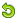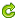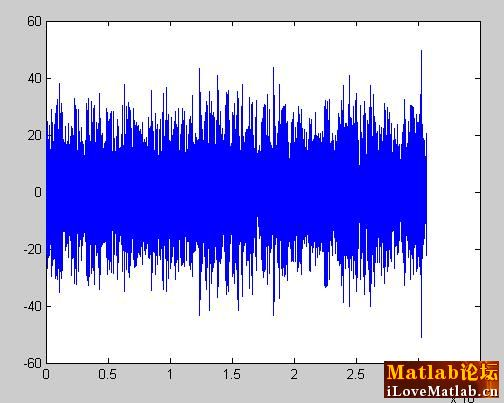展开全文• rms 计算作为向量、矩阵或离散值（标量）列表提供的值的均方根 (RMS)。 如果输入是矩阵，rms 返回一个行向量，其中包含每列的 RMS。 David Feldman 提出了以下更简单的函数定义： RMS = sqrt(mean([varargin{:}].^...matlab
• 今天的作业是求两幅图像的MSE、SNR、PSNR、SSIM.代码如下：clc;close all;X = imread('q1.tif');% 读取图像Y=imread('q2.tif');figure;% 展示图像subplot(1, 3, 1); imshow(X); title('q1');subplot(1, 3,...
• 实现matlab编程实现关于图像处理的一些简单的操作，加深对图像处理后图像性能评估的理解:  clc; close all; X = imread('1.jpg'); X=rgb2gray(X); Y=X; Y = imnoise(Y, 'salt &amp; pepper');%添加椒盐...图像处理 深度学习
• 本程序是基于MATLAB软件下的电压暂降时，如何求其均方根值曲线的程序
• MSE均方误差，用来检测模型的预测值与真实值之间的偏差，值越大表示预测效果越差，可在程序中通过程序名直接调用。适用于初学者学习测试matlab MSE
• 求取二组信号的均方根误差，程序很简单，一看就明白，直接输入二组信号即可求出均方根误差。matlab
• 时域计算代码相对简单，下列代码主要来自求助matlab时域分析程序-峰值、均方根、裕度、峭度_百度知道。不过原文中求“峭度因子”的方法不对，即“Kr = sum(y.^4)/sqrt(sum(y.^2))）”，求峭度直接用函数kurtosis就行...时域 信号处理 MATLAB
• 频域特征值5个——重心频率、均方频率、均方根频率、频率方差、频率标准差 信息熵特征值3个——功率谱熵、奇异谱熵、能量熵 （其他相关特征若干——样本熵等熵特征值、后续还会补充其他常用、不常用的特征指标） ...matlab 特征提取 时域特征 频域特征 重心频率
• matlab均方误差的代码RNN_XOR 该MATLAB代码处理了由RNN（递归神经网络）解决的XOR问题。 Sigmoid函数用于每个节点上的激活功能。 序列长度为两个，例如...误差的均方根用作损失函数。 反向传播算法是从pdf文件导出的。
• 基于UKF的匀速直线运动仿真及误差分析，提供了详细的基于matlab代码和运行结果分析UKF
• 残差平方和（SSE） 计算公式： 代码实现： sse = sum((YReal - YPred).^2); 均方误差（MSE） 计算公式： 代码实现： mse = sqrt(sum((YReal - YPred).^2))./2;...代码实现： ...代码实现 ...均方根误差（Rmatlab 机器学习
• 如下： tempdata=(true_y-predict_y).^2; tempdata2=(true_y-mean(true_y)).^2; r2=1 - ( sum(tempdata)/sum(tempdata2) ); sqrt(mean((predict_y-true_y).^2));matlab
• -------------------------------------------------------------------------------------------------------- 3、均方根误差: 均方根误差是均方误差的算术平方根 ----------------------------------------------...卡尔曼滤波 STM32 ADC 均方根
• （参考百度百科） 计算公式： 代码 % By lyqmath % DLUT School of Mathematical Sciences 2008 % BLOG：http://blog.sina.com.cn/lyqmath function [PSNR, MSE] = psnr(X, Y) % 计算峰值信噪比PSNR、均方根误差MSE...
• Root-mean-square energy 均方根能量 均方根能量表达的是一帧内所有样本点的一个综合信息 也能表达响度的信息，不过没有AE那样对异常敏感 应用：音频分割、音乐流派分类 Zero crossing rate 过零率 过零率是信号...信号处理 深度学习 机器学习 python
• rmse matlab代码

2021-04-21 10:49:45
[2 4],:,index).^2);...于是,在 Matlab 软件包中做线性多元回归,写一个 M—文件 opt_reg...Matlab 实现多元回归实例 实现多元回归 多元回归实例(一)一般多元回归一般...(x,y,'purequadratic') 在Matla...
• 做出上述三种信号的均方根图谱,功率图谱,以及对数均方根图谱4．用IFFT傅立叶反变换恢复信号，并显示恢复的正弦信号时域波形图源程序%************************************************************...
• 我们实现了一个线性回归模型分类器，该分类器使用年龄，性别和ECG前导信号的均方根作为特征。 这个简单的示例说明了如何为Challenge设置MATLAB条目的格式。 但是，它的设计得分不高（或更准确地说，设计得不好），......

matlab均方根代码matlab 订阅